Tutorial 14 – Openpyxl and read data from excel

Welcome to the fourteenth tutorial of Selenium-Python series! In this tutorial, we will study about Openpyxl and how to read data from excel.

What you will Learn:
• What is the need to read and write excel files
• Install openpyxl using pip
• Read cell value from excel file
• Count of rows and columns
• Write in a cell
• Custom function/Re-usable file
• Read cell value by column index
• Read cell value by column name
• Optimizing code
• Total number of rows and columns in the sheet using custom function
• Read all the row values
• Read all the column values by column name
• Read all the column values by column index
• Conclusion

What is the need to read and write excel files
In testing we have test cases that contain test data. An excel file is generally used to store the ‘Test Data’. There are 2 types of data:
a) Data that changes frequently (example, data such as username, password), this data is regular test data. To store this type of data, we use excel file.
b) Data that does not change frequently (example, url, page title, xpaths). To store this type of data, we use properties file.

Install openpyxl using pip
To read & write excel file, we use openpyxl. We use pip (package installer for Python) to install openpyxl.
See figure 1, goto command prompt & run ‘where pip’ to find out the location of pip installer in your machine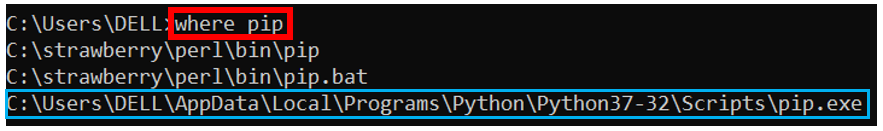Figure 1

cd to the location, see below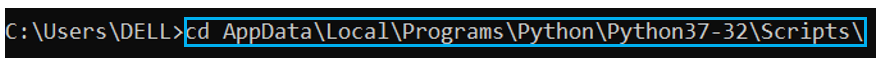Figure 2

Run ‘pip install openpyxl’ command, see belowFigure 3

After successful openpyxl installation, run the same command ‘pip install openpyxl’, you should get beow message ‘Requirement already satisfied’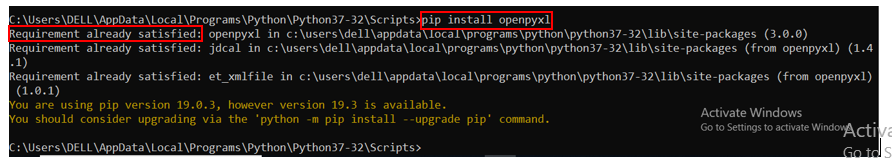Figure 4

Read cell value from excel file
Let us create an excel file having some test data, see below. Rename the worksheet as ‘TestData’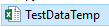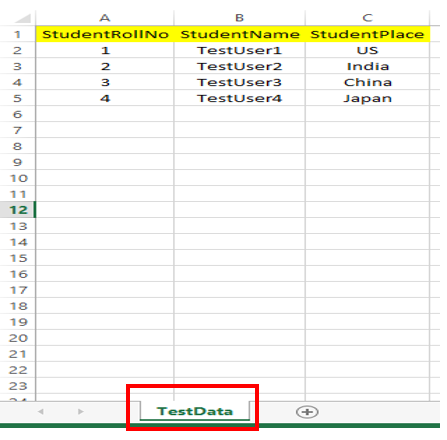Figure 5
Close the excel. To read from excel file, we need to import openpyxl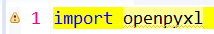Figure 6

Now give the path of the excel file, see below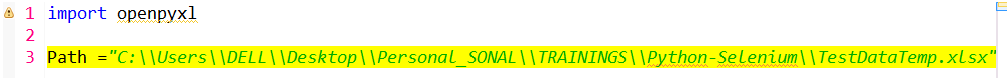Figure 7

Now we have to create a reference to this excel workbook file. Let ‘wb’ the name of the reference, see below. This reference is pointing to the path of .xlsx file. So this will load the workbook.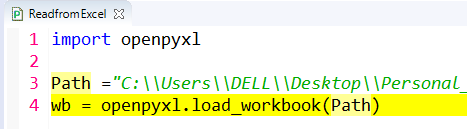Figure 8

Next, we have to read the data from the sheet, so we have to write over here, see line 5 below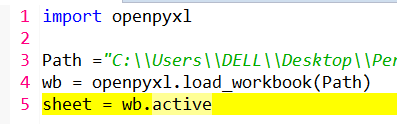Figure 9

Next, suppose we have to read the data ‘TestUser2’. The data is read using row index & column index. The row index & column index starts from 1,1 (StudentRollNo).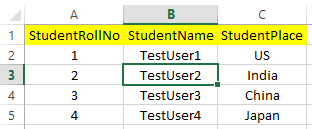Figure 10

Similarly, the ‘TestUser2’ row index is 3 & column index is 2. So read this data, see line 6 below (ensure you have closed the excel). See the o/p, ‘TestUser2’ gets printed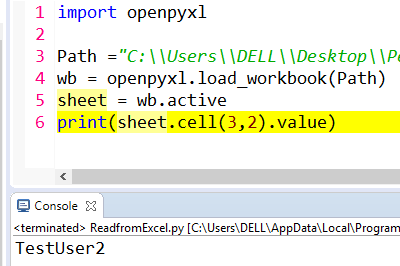Figure 11

Count of rows and columns
To count the number of rows & columns, see lines 7 and 8 below. So the number of rows is printed as 5 and number of columns as 3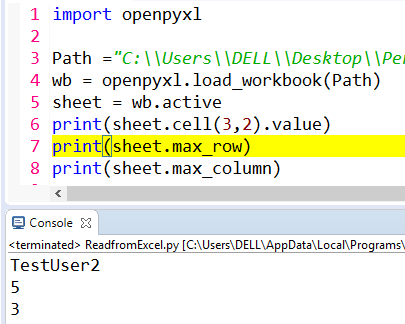Figure 12

Write in a cell
Now suppose we want to write the value ‘TestUser19’ in row index 3 & column index 2 viz we want to replace ‘TestUser2’ with ‘TestUser19’Figure 13

To do that, write lines 10, 11 and 12 as shown below. In line 10, we are capturing the cell(3,2) in a variable. In line 11, we are initializing the variable with a new value. In line 12 we are saving the workbook.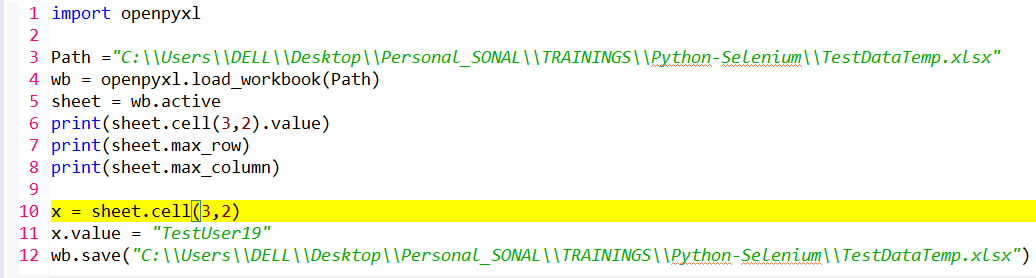Figure 14

When you run the program, the new value is seen in the cell, see below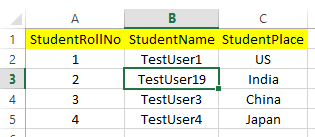Figure 15

In a similar way you can change the row and column indices & accordingly you can read or write the excel.

Custom function/Re-usable file
Now, instead of making these methods, we will simply make a re-usable custom file which we can integrate to any project or framework later. We will require these functions later as well. So we will create a class & make functions into it. To do that, let us first comment the lines 3-12, see belowFigure 16

Let us create a class readWriteExcel. We will make functions into it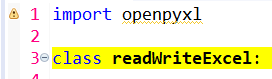Figure 17

Let us make 2 global variables:
a) wb (workbook that can be accessed by all the functions in the class)
b) excelFilePath
The advantage of creating global variables is that we don’t have to create these references again and again inside each function. Let these be initiated to ‘None’, see below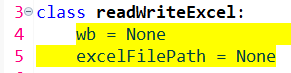Figure 18

We will now create a file initialization function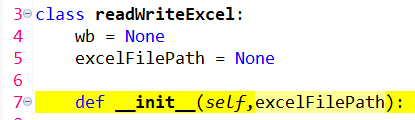Figure 19

We will next initialize the ‘wb’ & ‘excelFilePath’ variables inside the function, see below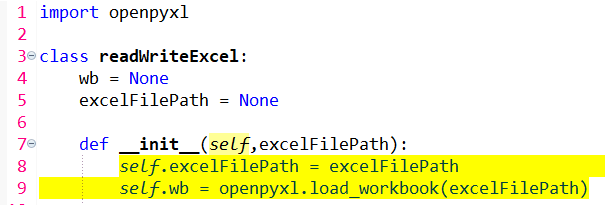Figure 20

Again, we made the 2 global variables so that we don’t have to give the excel file path into every function we make.

Read cell value by column index
Create a function readByColIndex that will accept 3 parameters: sheetName, rowIndex, colIndex, see below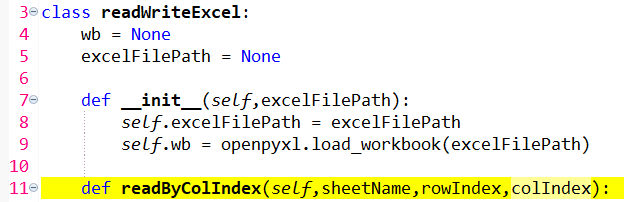Figure 21

See line 12 below. We have to give the sheetname viz sheet = self.wb[sheetName]. We will pass the sheetName when we call this function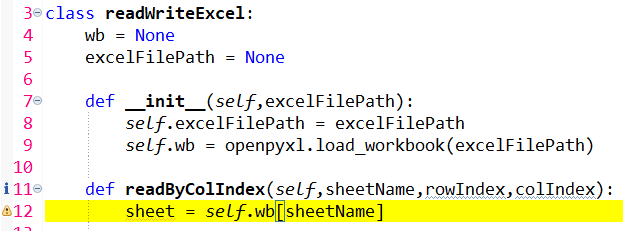Figure 22

Now, from this sheet, we would like to return the value of cell based upon the rowIndex & colIdex that we will pass while calling this function, see line 13 belowFigure 23

Now, instead of calling this function in the same module, let us create another module & we will than call this function by importing this class. So lets create another module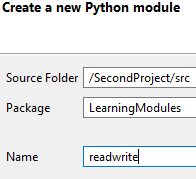Figure 24

See below. From the ‘ReadFromExcel.py’ module, we are importing the class ‘readWriteExcel’Figure 25

Now, re-look at ‘readWriteExcel’ class below. The init function accepts the ‘excelFilePath’ parameter. What this means is that, when we create an object of ‘readWriteExcel’ class, we have to pass ‘excelFilePath’ in the constructor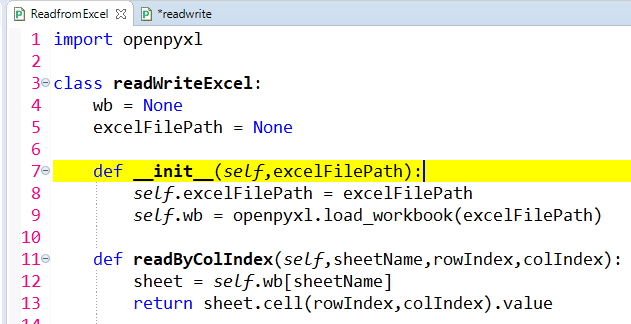Figure 26

So coming back to ‘readwrite’ module below, we have created an object of ‘readWriteExcel’ class and in the body of the constructor, we are passing ‘excelFilePath’.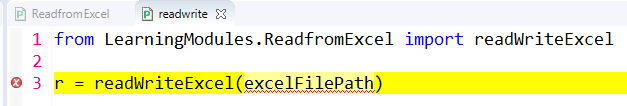Figure 27

So we will mention the file path as seen below. So what this essentially means is that, when we run the ‘readwrite’ module, an object of ‘readWriteExcel’ class would be constructed that would point to the excel file path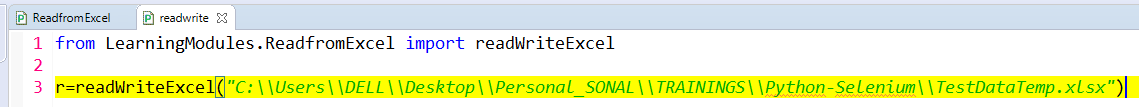Figure 28

To call the ‘readByColIndex’ function, we simply write below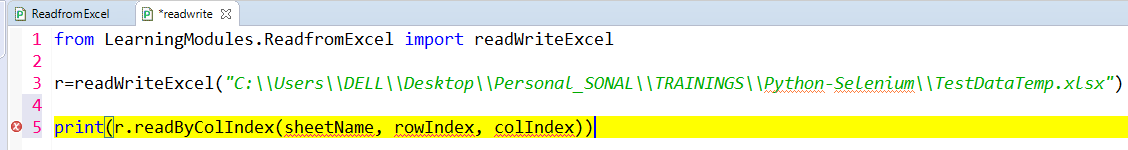Figure 29

Now see below, the sheetname is ‘Testdata’ and the col index of ‘Japan’ is 3 while row index is 5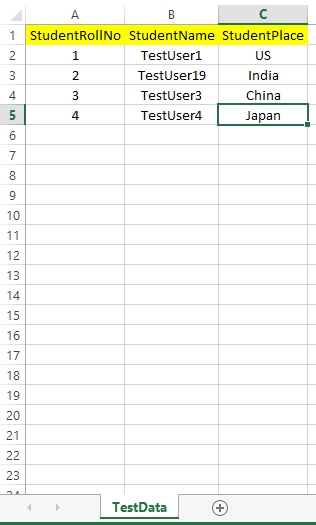Figure 30

Close the excel. Run the program, ‘Japan’ gets printed, see below. This is how we read data by column index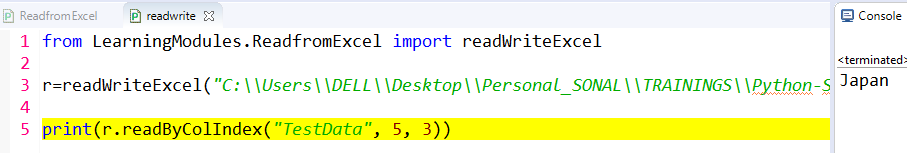Figure 31

Read cell value by column name
To read cell value by column name, we will create another function, see below. In line 15, we write ‘colName’ instead of ‘colIndex’. In line 17, we are initializing ‘colIndex’ to 1. We than start a while loop. What we are trying to say in line 18 is that:
We will check if the value of row number=1 & column number = ‘colIndex’ (that we already initialized to 1) is NOT equal to blank. We will than use the ‘if’ statement (line 19). What it means is that, if the colName (that we pass when we call this function) is equal to the cell value, than it will simply break (line 20). If the colName (that we pass when we call this function) is NOT equal to the cell value, than it will increase the colIndex by 1 (line 21). After increasing the colIndex, we will again check using the while loop if the colName (that we pass when we call this function) is equal to the cell value, than it will break, otherwise again the colIndex will be incremented by 1 & process repeats. At the end, it returns us the cell value (line 22)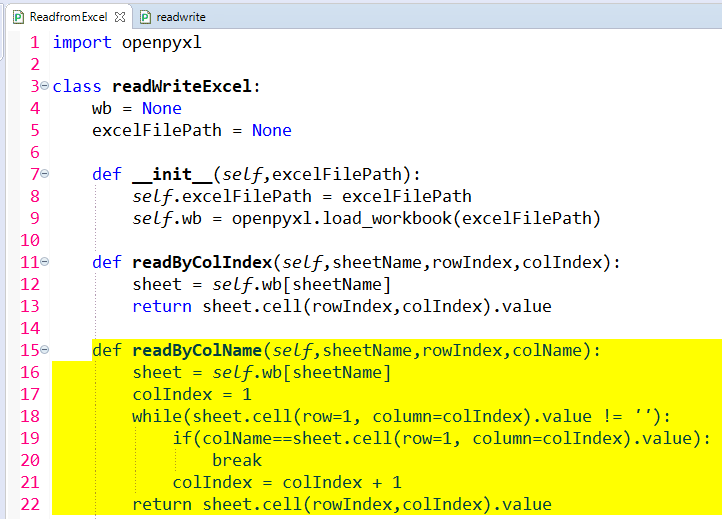Figure 32

See below. if we want to read a cell value in the column name ‘StudentPlace’, we know that its row index is 3.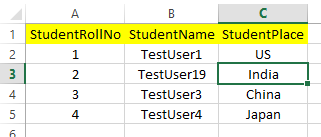Figure 33

So see line 6 below, it prints ‘India’ in console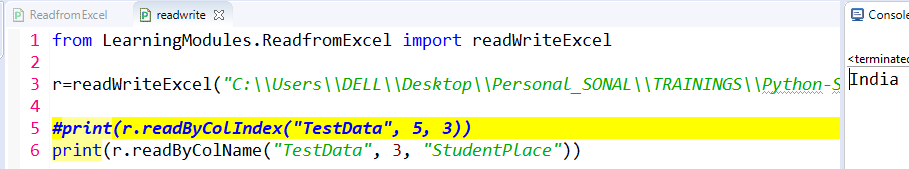Figure 34

Below is how the logic is working: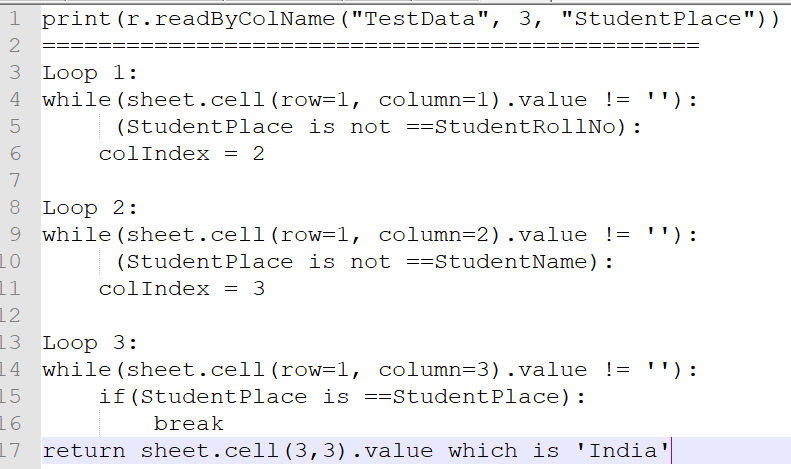Figure 35

So this is how we read the cell value by name.

Optimizing code
Now if you see below, we are repeating lines 12 & 16 again and again in every function. This repetition is not needed.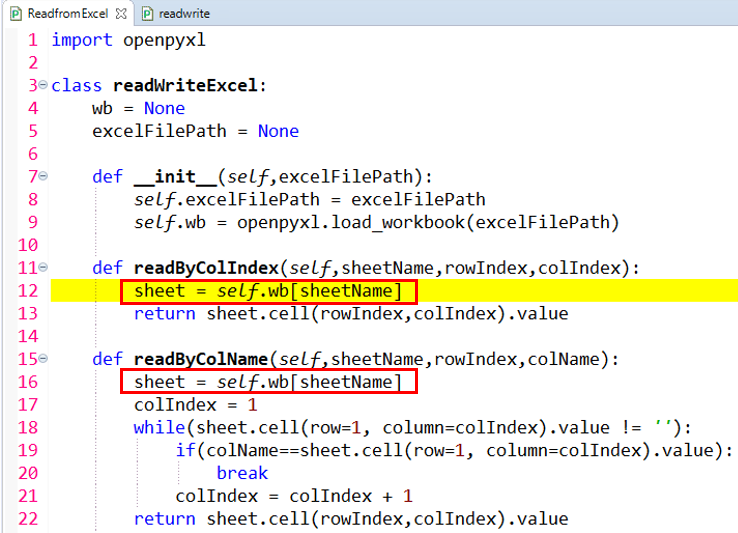Figure 36

Python has got one functionality that if we want to use the global variable inside the function, we will simply use the keyword ‘global’. So we will do these changes in above figure:
---line number 7, change ‘excelFilePath’ to ‘FilePath’
---write ‘global wb’ & ‘global excelFilePath’
---line number 8, instead of "self.excelFilePath = excelFilePath" we will write "excelFilePath = FilePath"
---Remove ‘self’ from lines 12 & 16 in above figure
So see below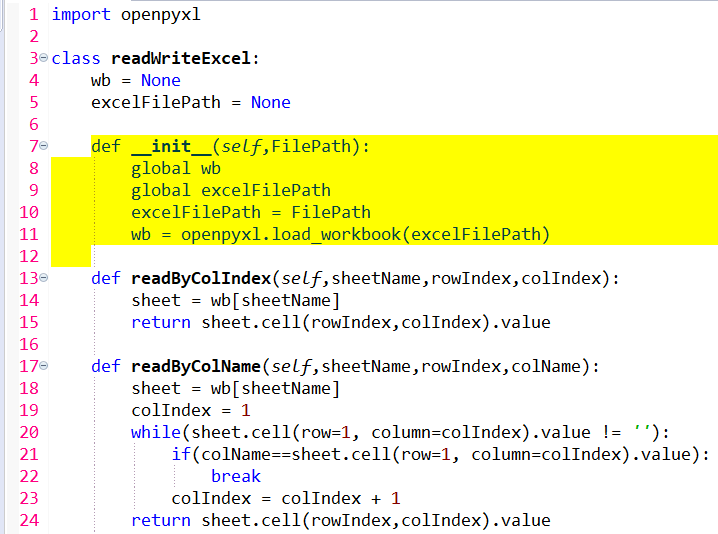Figure 37

When we run below program, we still get the same o/p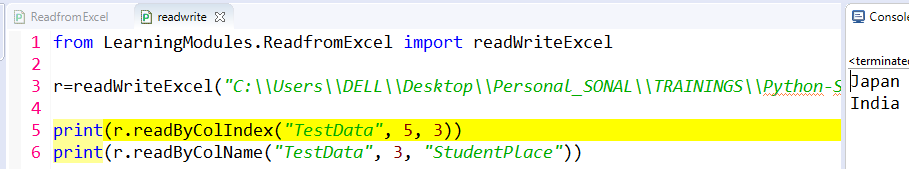Figure 38

This is how we make the global variables work inside the function.

Total number of rows and columns in the sheet using custom function
We create below 2 functions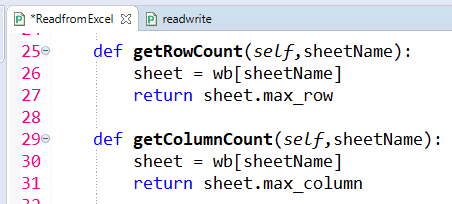Figure 39

So we can call these functions as seen below (matches with excel)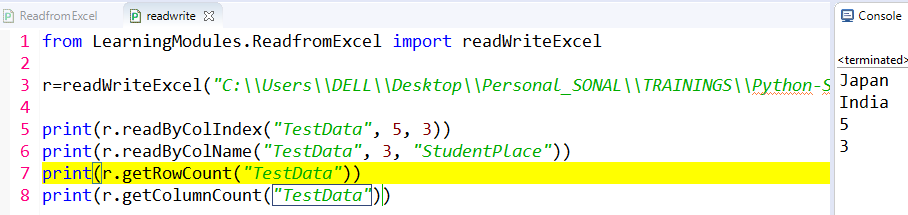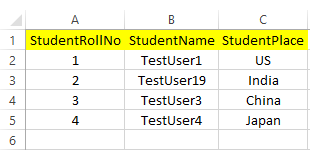Figure 40

Let us say we want to read all the row values (example: 1, TestUser1, US), than how do we achieve that?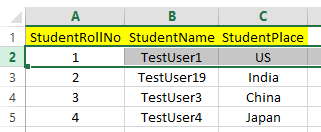Figure 41

The below code does that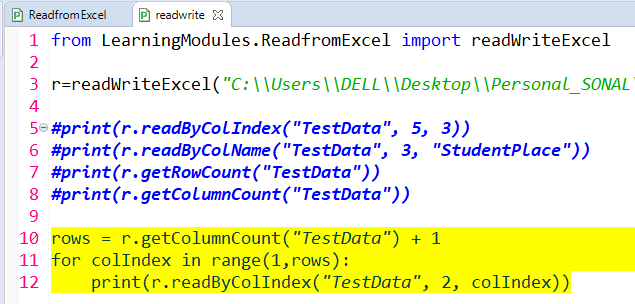Figure 42

When we run this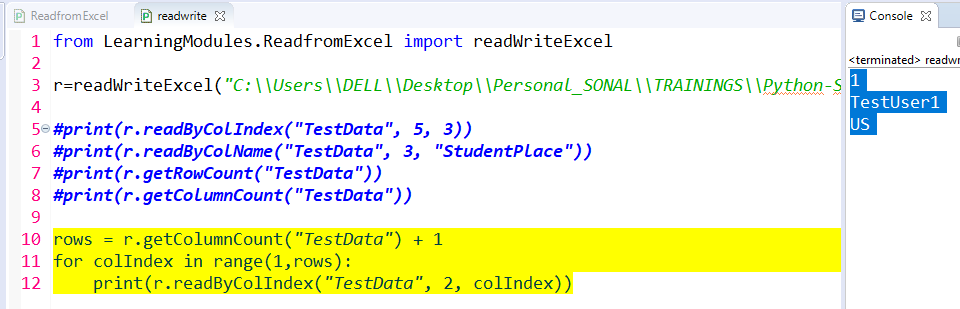Figure 43

Below will explain above code’s logic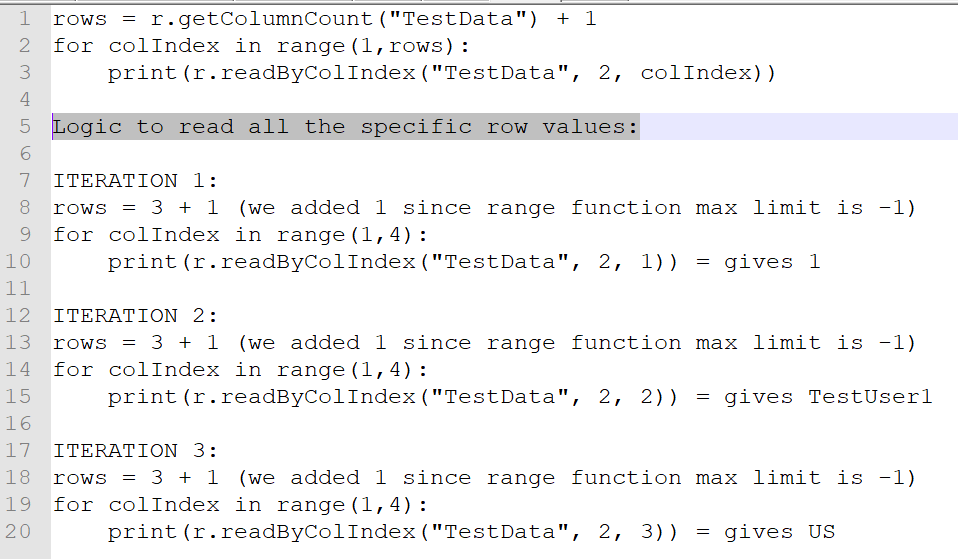Figure 44

Read all the column values by column name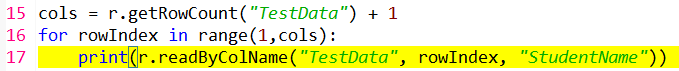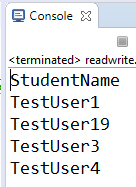Figure 45

Below is logic explanation: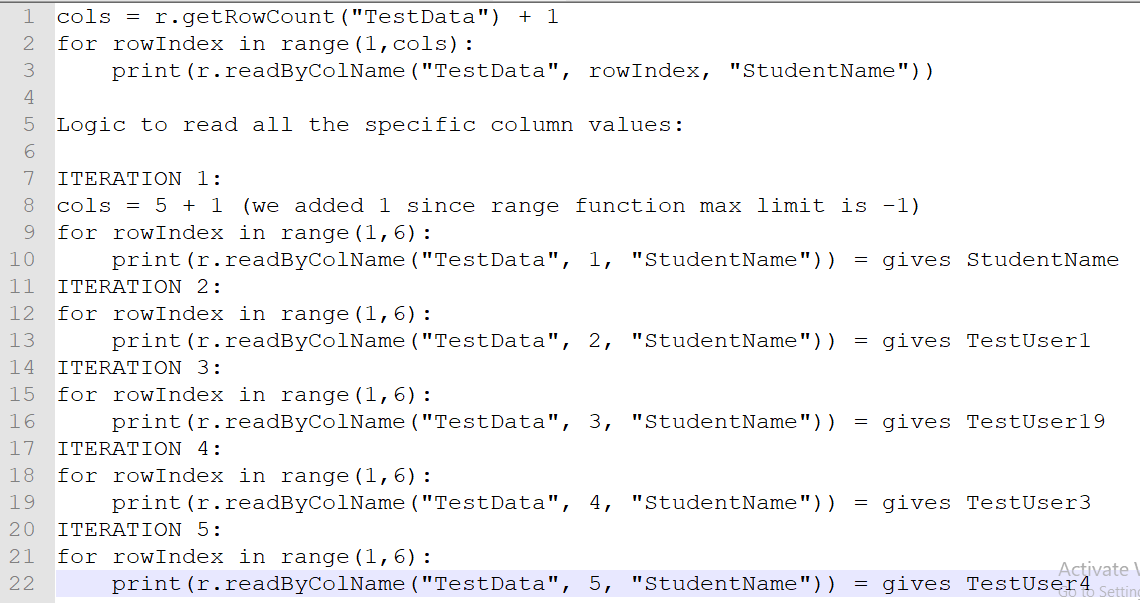Figure 46

Read all the column values by column index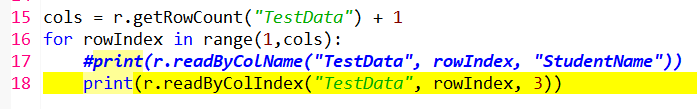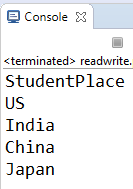Figure 47

Below is logic explanation: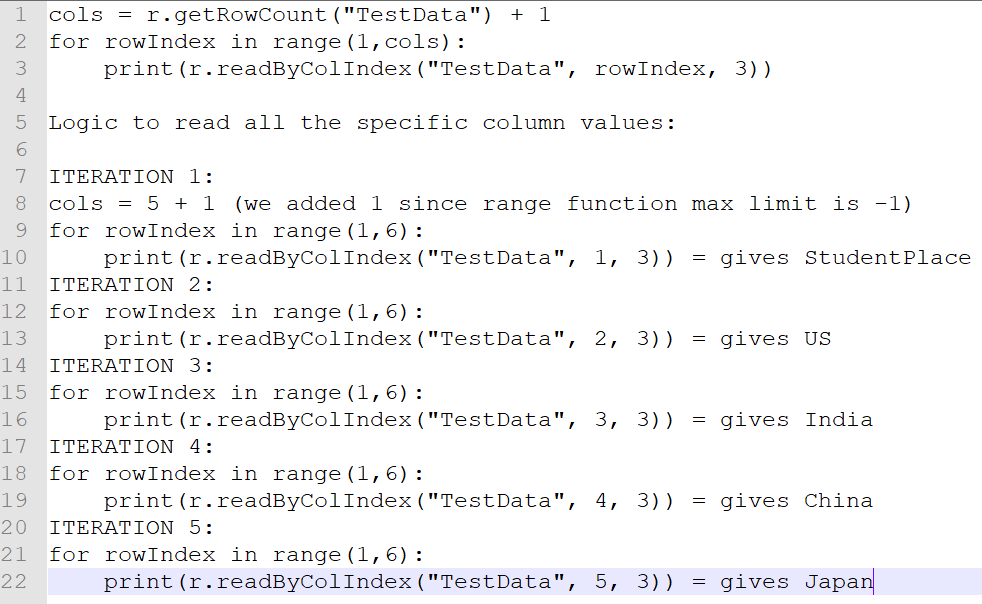Figure 48

Conclusion
In this tutorial we have studied lot of topics around reading data from excel using openpyxl utility. Thank you!